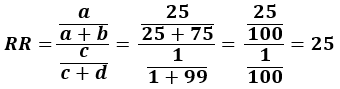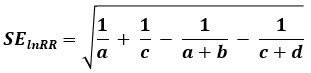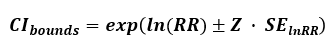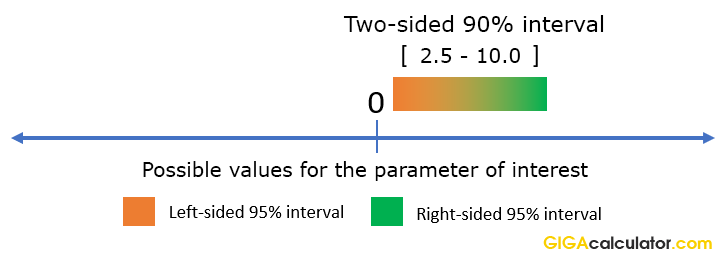# Relative Risk Calculator

Use this relative risk calculator to easily calculate relative risk (risk ratio), confidence intervals and p-values for relative risk between an exposed and a control group. One and two-sided intervals are supported for both the risk ratio and the Number Needed to Treat (NNT) for harm or benefit.

%

### Calculation results

1.009091 Relative Risk (Risk Ratio) 95% Confidence Interval 95% Left-Sided Interval 95% Right-Sided Interval P-value Z-score Number Needed to Treat (Harm) 95% NNT Confidence Interval
Share calculator:

Embed this tool:
get code

## Using the relative risk calculator

This relative risk calculator allows you to perform a post-hoc statistical evaluation of a set of risk data when the outcome of interest is the change in relative risk (the risk ratio) or the absolute risk difference (ARR) between an exposed/treatment group and a control group. To use the tool you need to simply enter the number of events and non-events (e.g. disease and no disease) for each of the two groups. You can select any level of significance you require for the confidence intervals.

The risk ratio calculator will output: relative risk, two-sided confidence interval, left-sided and right-sided confidence interval, one-sided p-value and z-score, the number needed to treat to achieve the benefit for a single person (NNT Benefit) or number of people that need to be exposed for one negative outcome to occur (NNT Harm). A two-sided confidence interval for NNT is also presented.

## What is relative risk and what is a risk ratio?

The two are synonyms and denote the relative difference in risk between an exposed group and a control group, or a treatment group and a control group, depending on context. Both express the ratio of the probability of an event occurring (e.g. developing a disease or condition, being injured, dying, etc.) in an exposed group to the probability of the event occurring in a comparison, non-exposed group: RR = P(event when exposed) / P(event when not exposed). This is what is computed by this risk ratio calculator.

Practical example: if we take smokers and risk of lung cancer as an example, if we know that from the exposed group (smokers) 25 developed some kind of lung cancer and 75 remained cancer free, while in the non-smokers 1 person developed lung cancer and 99 remained cancer-free, what is the relative risk of smokers versus non-smokers?

If we denoted the smokers who developed cancer with a, those who did not with b, the non-smokers who developed cancer with c and those who did not with d the formula and solution will look like so:This is the result you would get using the relative risk calculator, too. We can conclude that a smoker will have a relative risk 25 times higher than a non-smoker to develop lung cancer, which is not far from truth (according to US CDC data the true risk is between 15 and 30 times). Relative risk should not be confused with absolute risk, which in this case is 25/100 or 25%, or 1 in 4.

## Relative risk vs Odds Ratio vs Hazard Ratio

Relative risk and risk ratios (probability ratios) are different from odds ratios, although they might be close in certain cases. Even though odds ratios have more practical applications, relative risk is arguably a more intuitive measure of effectiveness and so has its applications in fields like epidemiology, clinical research including randomized control trials, as well as cohort analysis and longitudinal observational studies.

One advantage of odds ratios is, however, that they are invariant to the variable of interest: they will vary proportionally in both effect directions while a risk ratio is skewed and can produce highly differing results when looking at the complimentary proportion instead. As an extreme example of the difference between risk ratio and odds ratio, if action A carries a risk of a negative outcome of 99.9% while action B has a risk of 99.0% the relative risk is approximately 1 while the odds associated with action A are more than 10 times higher than the odds in doing B (1% = 0.1% x 10, odds ratio calculation, relative risk calculation).

The highly disparate results are due to the definition of risk based on the negative events. If we define risk by using the positive outcome instead, we get a relative risk of 0.10 which has a much better correspondence with the odds ratio. Relative risk is usually the preferred metric, but risk must be precisely defined to avoid confusion.

Relative risk is also sometimes confused with hazard ratios, but they are even more different as metrics than odds ratios and risk ratios. The outputs of this relative risk calculator should not be confused with odds ratios or hazard ratios.

## Number Needed to Treat (NNT) for harm or benefit

One of the results from this calculator is the Number Needed to Treat, abbreviated NNT. It is a useful representation of the effectiveness of a treatment in providing benefit or in the harmfulness of exposure to a given condition or chemical. It represents the number of people that need to be treated in order for 1 person to benefit, or the number of people that need to be exposed for 1 person to experience a negative event. Mathematically it is simply the reciprocal of the absolute risk difference.

The interpretation of the number if fairly straightforward: a treatment that will lead to one saved life for every 10 patients treated is clearly better than a competing treatment that saves one life for every 50 treated. According to Cook & Sacket (1995)  "It has the advantage that it conveys both statistical and clinical significance to the doctor". It is used in single analyses and meta analyses, alongside its confidence intervals  which are also available in our relative risk calculator above.

To avoid negative values and unintuitive interpretations, two notations are introduced: number needed to treat for harm (NNTH) and number need to treat for benefit (NNTB) and their alternatives NNT(benefit) NNT(harm) which seem more widely adopted for now. Similarly if the NNT interval covers zero it is represented a bit differently to denote that it covers infinity and the two bounds have the labels (harm) and (benefit) attached to them to make it explicit what they refer to, again, avoiding nonintuitive negative values.

With that said, some people incorrectly interpret the NNT to mean that this number of people will not have any benefit (or harm) while all the benefit (or harm) accrues to one individual out of the NNT number. This is obviously incorrect, since NNT is an average related to the relative risk, not a dichotomous outcome. In fact, most studies do not provide enough information to estimate the average change of relative risk, instead they make claims about the change of the average relative risk.

## What is a risk ratio confidence interval and "confidence level"

Note that the relative risk calculator produces confidence intervals for risk ratios. A confidence interval is defined by an upper and lower boundary for the value of a variable of interest and it aims to aid in assessing the uncertainty associated with a measurement, usually in experimental context. The wider an interval is, the more uncertainty there is in the relative risk estimate. Every confidence interval is constructed based on a particular required confidence level, e.g. 0.09, 0.95, 0.99 (90%, 95%, 99%) which is also the coverage probability of the interval. A 95% confidence interval (CI), for example, will contain the true value of the risk ratio 95% of the time (in 95 out of 5 similar experiments).

Simple two-sided confidence intervals are symmetrical around the observed risk ratio, but in certain scenarios asymmetrical intervals may be produced. In any particular case the true value may lie anywhere within the interval, or it might not be contained within it, no matter how high the confidence level is. Raising the confidence level widens the interval, while decreasing it makes it narrower. Similarly, larger sample sizes result in narrower intervals, since the interval's asymptotic behavior is to be reduced to a single point.

## Standard error and confidence interval formula for risk ratios

The standard error of the log risk ratio is known to be:Accordingly, confidence intervals are calculated using the formula:where RR is the calculated risk ratio (relative risk), SElnRR is the standard error for the log risk ratio and Z is the score statistic, corresponding to the desired confidence level. This is the equation used in our risk ratio calculator. The Z-score corresponding to a two-sided interval at level α (e.g. 0.90) is calculated for Z1-α/2, revealing that a two-sided interval, similarly to a two-sided p-value, is calculated by conjoining two one-sided intervals with half the error rate. E.g. a Z-score of 1.6448 is used for a 0.95 (95%) one-sided confidence interval and a 90% two-sided interval, while 1.956 is used for a 0.975 (97.5%) one-sided confidence interval and a 0.95 (95%) two-sided interval. Therefore it is important to use the right kind of risk ratio interval: more on one-tailed vs. two-tailed intervals.

## One-sided vs. two-sided intervals

While confidence intervals are customarily given in their two-sided form, this can often be misleading if we are interested if a particular value below or above the interval can be excluded at a given significance level. A one-sided relative risk interval in which one side is plus or minus infinity is appropriate when we have a null / want to make statements about a risk ratio value lying either above or below the top / bottom bound . By design a two-sided interval is constructed as the overlap between two one-sided intervals at 1/2 the error rate 2.

For example, if we have the two-sided 90% interval for relative risk spanning (2.5, 10), we can actually say that risk ratios less than 2.5 are excluded with 95% confidence precisely because a 90% two-sided interval is nothing more than two conjoined 95% one-sided intervals:Therefore, to make directional statements about relative risk based on two-sided intervals, one needs to increase the significance level for the statement. In such cases it is better to use the appropriate one-sided interval instead, to avoid confusion. Our relative risk calculator conveniently does the math for you.